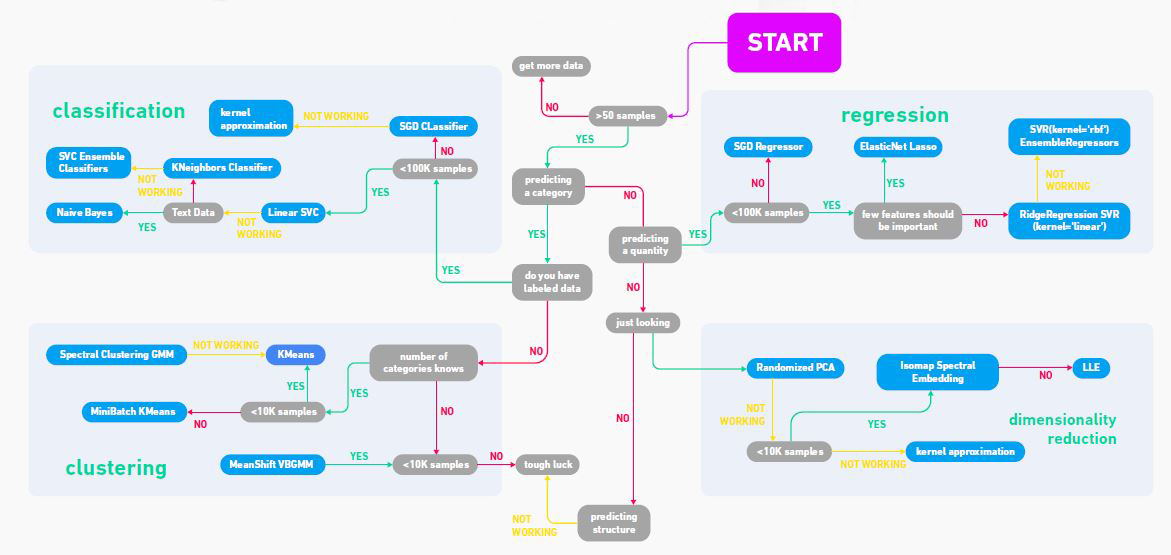Related Articles
Flowchart for basic Machine Learning models
• Last Updated : 05 Sep, 2020

Machine learning tasks have been divided into three categories, depending upon the feedback available:

1. Supervised Learning: These are human builds models based on input and output.
2. Unsupervised Learning: These are models that depend on human input. No labels are given to the learning algorithm, the model has to figure out the structure by itself.
3. Reinforcement learning: These are the models that are feed with human inputs. No labels are given to the learning algorithm. The algorithm learns by the rewards and penalties given.

The algorithms that can be used for each of the categories are:

The machine learning functions and uses for various tasks are given in the below table. To know more about the Algorithms click here.

The flowchart given below will help you give a rough guide of each estimator that will help to know more about the task and the ways to solve it using various ML techniques.My Personal Notes arrow_drop_up